# Basic Geometry: Rules & Formulas

Lesson Transcript
Instructor: Stephanie Matalone

Stephanie taught high school science and math and has a Master's Degree in Secondary Education.

Explore the world of geometry, a mathematical field that studies space, dimensions, and shapes. Learn about area, surface area, and volume. Discover the formulas to calculate the measurements of two-dimensional and three-dimensional shapes. Updated: 11/27/2021

## Geometry

In its simplest form, geometry is the mathematical study of shapes and space. Geometry can deal with flat, two-dimensional shapes, such as squares and circles, or three-dimensional shapes with depth, such as cubes and spheres.

Before diving into two-dimensional and three-dimensional shapes, consider the basic geometric objects that create these shapes: points, lines, line segments, rays, and planes.

A point is represented by a dot and shows a location in space. A line is a set of straight points that extends forever in both directions as depicted by arrows on both ends. Rays are lines that end on one side. Line segments end on both sides. Planes are surfaces that extend forever in all directions.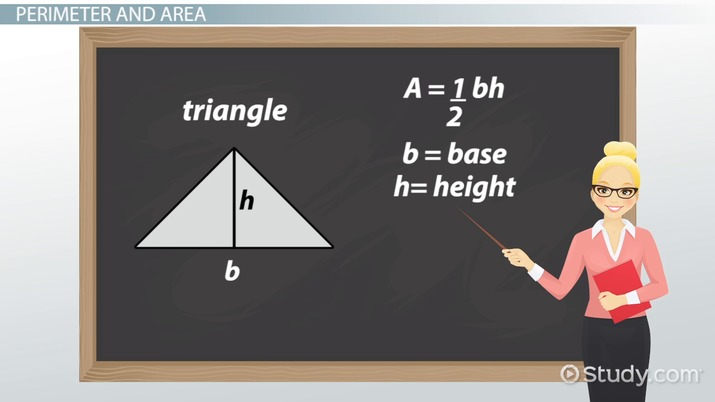An error occurred trying to load this video.

Try refreshing the page, or contact customer support.

Coming up next: What is a Plane in Geometry? - Definition & Examples

### You're on a roll. Keep up the good work!

Replay
Your next lesson will play in 10 seconds
• 0:00 Geometry
• 0:50 Two-Dimensional Shapes
• 1:30 Perimeter And Area
• 4:05 Three-Dimensional Shapes
• 4:35 Surface Area And Volume
• 7:15 Lesson Summary
Save Save

Want to watch this again later?

Timeline
Autoplay
Autoplay
Speed Speed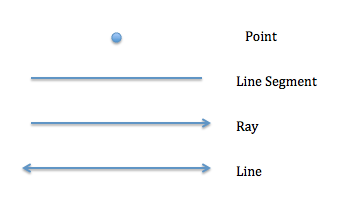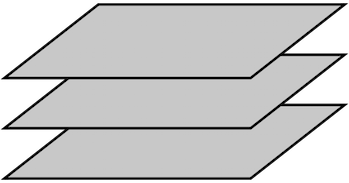## Two-Dimensional Shapes

Two-dimensional objects only have two dimensions: length and width.

Polygons are two-dimensional shapes made up of line segments. In order to be considered a polygon, a set of line segments must be closed, meaning each line segment meets up with another line segment. Because of this requirement, squares and triangles are considered polygons, while a circle is not a polygon.

Squares are polygons made of four line segments, where each segment is the same length. Rectangles are also made of four line segments, where two parallel segments are equal length, and the other two parallel segments are equal length. Triangles are polygons with three line segments that can be equal length, but don't need to be.

## Perimeter and Area

Perimeter is a commonly calculated measurement with two-dimensional shapes in geometry that adds the length of a polygon's line segments. Perimeter calculations are for different applications, including finding how much fencing to put up around your yard.

As illustrated, perimeter calculations are essentially the same for the different shapes: the length of each separate line segment must be added together. For example, if one side of a square, a, measures 12 inches, then using the formula for a square's perimeter, 4a, p is 4 times 12, which equals 48 inches.

The formulas for finding the perimeter of a rectangle and a triangle also require finding the sum of the length of the sides:

Perimeter of a rectangle = (2 * length) + (2 * width)

The perimeter of a triangle = a + b + c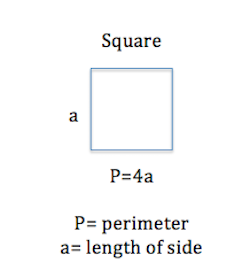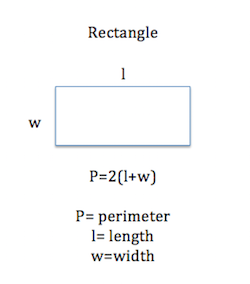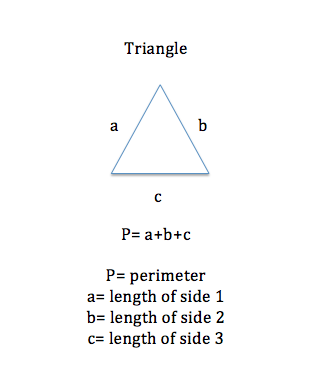The perimeter measurement of a circle is known as circumference. The circumference of a circle is found by using diameter d (the distance across a circle), or radius r (the distance halfway across a circle), and pi, which is a ratio used in geometry that roughly equals 3.14.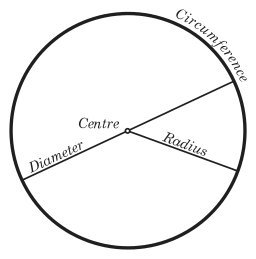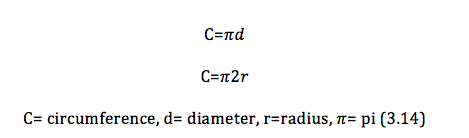Here's an example of how to calculate a circle's circumference. To find the circumference of a circle with a radius r of 4 meters, simply multiply 4 by 2 and by pi (3.14). The circumference of that circle would be approximately 25.12 meters. The circumference = 4 * 2 * 3.14

Area is the measure of the surface of an object. The area of a square, rectangle, triangle, and circle can be found using formulas. Area calculations are used, for instance, when people want to know the square footage of their homes.

The formula for the area of a square is A = l^2 (l = length of a side). The formula for finding the area of a rectangle is A = length * width. When finding the area of a triangle, the formula area = ½ base * height.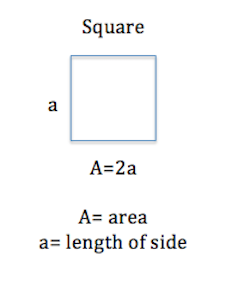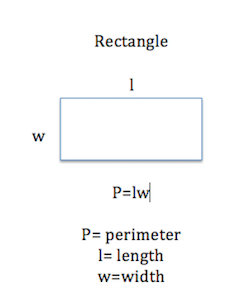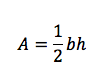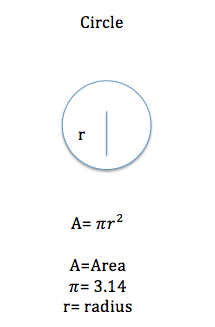As an example, to find the area of a triangle with a base b measuring 2 cm and a height h of 9 cm, multiply 1/2 by 2 and 9 to get an area of 9 cm squared. Area is ½ * 2 * 9 = 9.

The formula for the area of a circle A = pi * r^2. This means use 3.14 (for pi) times the radius-squared.

## Three-Dimensional Shapes

Unlike two-dimensional objects, three-dimensional objects have a third dimension, depth, and are, therefore, not flat. Cubes, spheres, and pyramids are examples of three-dimensional objects. A cube is an object made of six square sides. A sphere is an object shaped like a ball, where every point on the surface is the same distance from the center of the ball. A cylinder is another three-dimensional object like a can with two circular ends and curved sides.

To unlock this lesson you must be a Study.com Member.

### Register to view this lesson

Are you a student or a teacher?